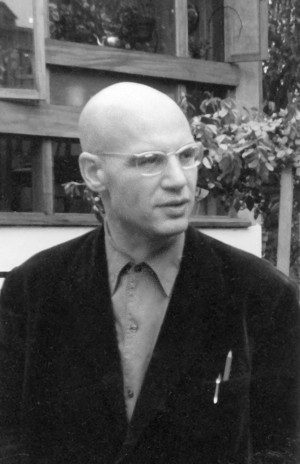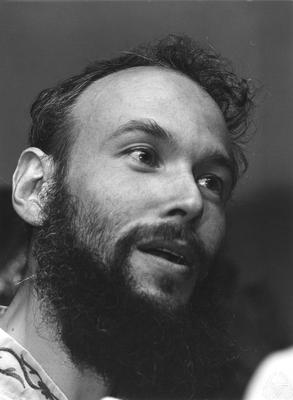# Étale cohomology and the Weil conjectures (Spring 2022)

We will review some important results in etale cohomology in the first third of this seminar, including the proof of the Weil conjectures except for the Riemann hypothesis. For the remainder of the seminar we will study Deligne's second proof of the Riemann hypothesis [D], following some simplifications of Laumon using the l-adic Fourier transform [L]. This material may be found in [KW], and the seminars [B], [C] cover similar content.

• Organizer: Caleb Ji
• When: Friday 5:00 pm - 6:00 pm
• Where: Math 528
• References:
[D] P. Deligne, La conjecture de Weil. II, Publ. Math. IHES 65 (1980), 137–252.
[L] G. Laumon, Transformation de Fourier, constantes d' équations fonctionelles et conjecture de Weil, Publ. Math. IHES 65 (1987), 131-210.
[KW] R. Kiehl and R. Weissauer Weil Conjectures, Perverse Sheaves and l’adic Fourier Transform 42 Springer-Verlag, Berlin, 2001.
[B] Michigan seminar
[C] Stanford seminar
• SOME notes from the seminar are here (not everything covered in this seminar was written up).

#### Schedule

Feb 4
Caleb Ji
Introduction to étale cohomology
We will briefly review the definition of étale cohomology and discuss its basic properties. Then we will go through some basic computations, including the étale cohomology of curves and of number fields.
Feb 11
Caleb Ji
The proper base change theorem
We will discuss the proof of the proper base change theorem, which uses important ideas including dévissage, algebrization theorems, and Artin approximation. Then we will discuss a few of the many consequences, such as the definition of cohomology with proper support.
Feb 18
Matthew Hase-Liu
Frobenius action, monodromy, and proof of the Riemann hypothesis for hypersurfaces
We first review the different Frobenii on $$\mathbb{F}_q$$-schemes, along with their actions on cohomology. We then make a quick foray into monodromy land to understand the category of lcc sheaves in a more geometric way - for instance, we will see a variant of Hurewicz theorem in the l-adic context. Using this monodromy-theoretic language, we then present Katz's proof of the Riemann hypothesis for hypersurfaces, which uses the method of continuity from complex analysis (as discussed in Prof. Phong's class).
Feb 25
Caleb Ji
Étale cohomology as a Weil cohomology and the Lefschetz trace formula
We will list and prove most of the results that make étale cohomology into a Weil cohomology: the cycle class map, Poincaré duality, the Künneth formula, and the Weak Lefschetz theorem. We will then see how this implies these results imply the Lefschetz trace formula and subsequently most of the Weil conjectures.
Mar 4
Caleb Ji
The Grothendieck trace formula
The Grothendieck trace formula is an important generalization of the Lefschetz trace formula from constant sheaves to constructible sheaves. It is a key component to Deligne's proof of Riemann hypothesis which we will cover later in this seminar. We will prove this theorem and see an application to exponential sums.
Mar 11
Caleb Ji
Weil sheaves and weights
We will introduce Weil sheaves, which allow us to extend the Grothendieck trace formula further.
Mar 18
Spring break
Mar 25
Caleb Ji
Weights and monodromy
We will introduce the concept of weights and prove an important semicontinuity theorem for them. Then we will discuss the sheaf-function correspondence and see how the results on weights give us bounds on the radius of convergence of relevant L-functions. We end by showing rank 1 Weil sheaves are $$\tau$$-pure, which allows us to define determinant weights.
Apr 1
Matthew Hase-Liu
Real sheaves and the $$\ell$$-adic Fourier transform
We begin by sketching a proof that all $$\tau$$-real sheaves are $$\tau$$-mixed, which will be one of the key steps in the proof of the Weil conjectures. Then, we will develop the basics of the $$\ell$$-adic Fourier transform over the affine line, closely mirroring the formalism of the classical Fourier transform over finite fields. This will ultimately give us a tool to "deform" the cohomology of a sheaf over the affine line.
Apr 8
Caleb Ji
The generalized Weil conjectures
The generalized Weil conjectures gives bounds on the eigenvalues of cohomology groups of lisse $$\overline{\mathbb{Q}_l}$$-sheaves. Restricting to the case of constant sheaves gives the Riemann hypothesis of the Weil conjectures. We will use the theory developed over the course of the seminar so far to give a proof of the generalized Weil conjectures, assuming a certain fact about perverse sheaves which will motivate our study of perverse sheaves in future weeks.
Apr 15
Caleb Ji
Perverse sheaves
We give an introduction to perverse sheaves. We will show perverse sheaves form a Noetherian and Artinian abelian category and describe how the simple perverse sheaves aris from intersection cohomology complexes. We end by giving an overview of various areas of math where perverse sheaves naturally arise.
Apr 22
Caleb Ji
The decomposition theorem
The first half of this talk will be devoted to examples and computations of perverse sheaves and intersection cohomology. In the second half, I will state the decomposition theorem and indicate how the generalized Weil conjectures can be applied to prove it.
Apr 29
No seminar
May 10 Note the date change!
Kevin Chang
Betti numbers of Hilbert schemes of points on a surface
I'll talk about Göttsche-Soergel's computation of the Betti numbers of Hilbert schemes of points on a surface using the decomposition theorem. Along the way, we'll review a bit of the geometry of Hilbert schemes of points, such as the natural stratification and the Hilbert-Chow morphism.